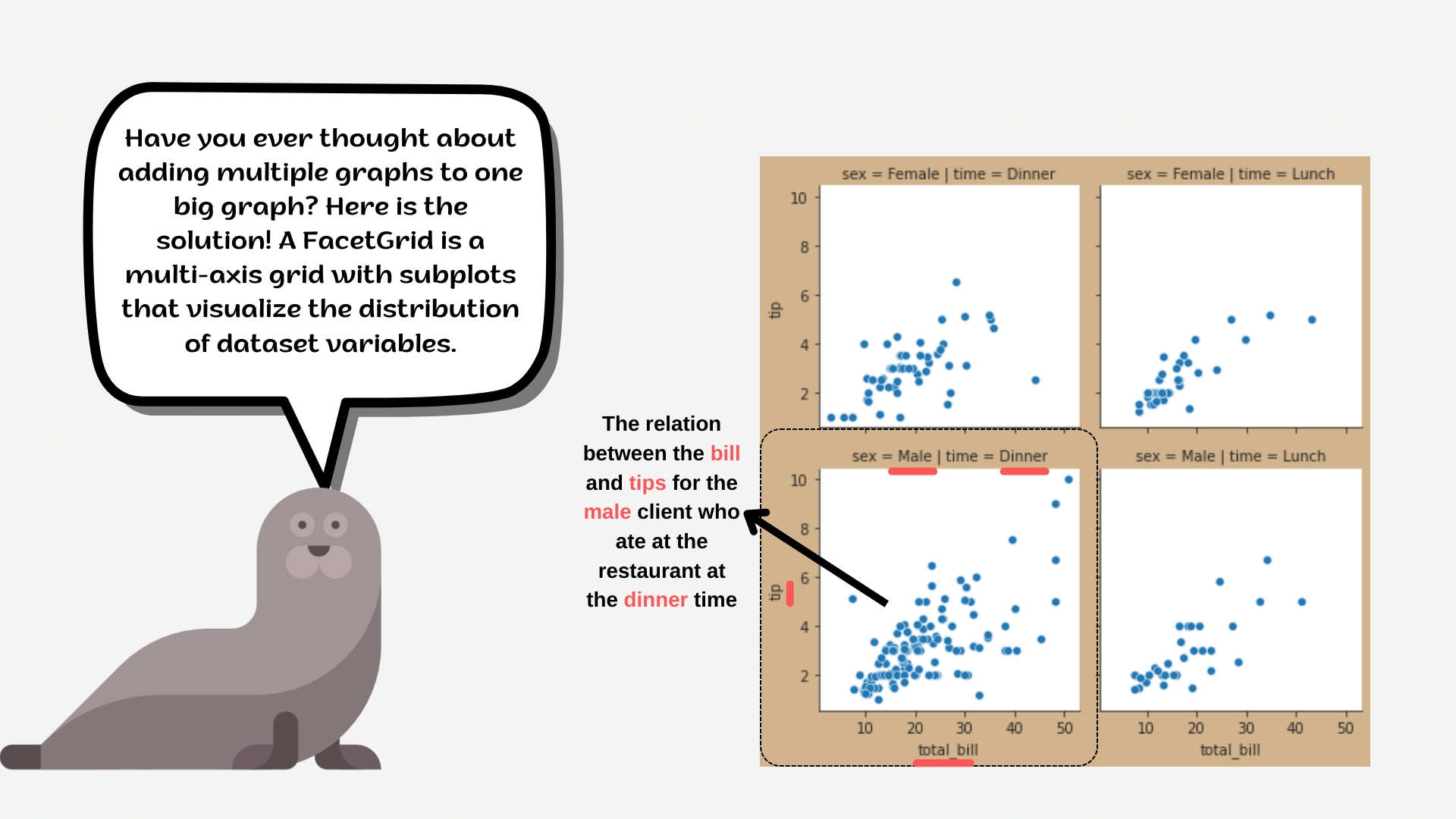Course Content

# Deep Dive into the seaborn Visualization

1. Light Start

2. Distributions of Data

3. Categorical Plot Types

4. Matrix Plots

5. Multi-Plot Grids

6. Regression Models

Deep Dive into the seaborn Visualization

##FacetGridThe time to learn the cool plots we were talking about!

A useful approach to exploring medium-dimensional data is by drawing multiple instances of the same plot on different subsets of your dataset.

`FacetGrid` object takes a DataFrame as input and the names of the variables that will form the grid's row, column, or hue dimensions. The variables should be categorical, and the data at each level of the variable will be used for a facet along that axis.1. Set the `'whitegrid'` style with the `'cornsilk'` `axes.facecolor`.
2. Create a FacetGrid variable `g`:
• Set the data for the `FacetGrid`;
• Set the `col` parameter equals the `'day'`;
• Set the `row` parameter equals the `'smoker'`;
• Set the `height` parameter equals `3`.

Implement the `.map()` function to build histplots:

• Create histplots;
• Set the `'olive'` color for the histplots;
• Add the `kde` parameter;
• Disable the `fill` parameter;
• Set the `binwidth` equals `4`;
• Display the plot.

Everything was clear?

Section 5. Chapter 1